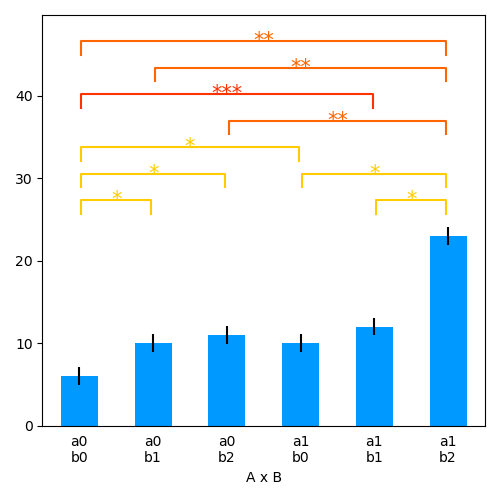# Repeated measures ANOVA

Based on 1.

```# Author: Christian Brodbeck <christianbrodbeck@nyu.edu>
from eelbrain import *

y = Var([7,  3,  6,  6,  5,  8,  6,  7,
7, 11,  9, 11, 10, 10, 11, 11,
8, 14, 10, 11, 12, 10, 11, 12],
name='y')
a = Factor('abc', repeat=8, name='A')
```

Fixed effects ANOVA (independent measures, 1 p. 24):

```print(test.ANOVA(y, a, title="Independent Measures"))
```

Out:

```Independent Measures

SS   df      MS          F        p
---------------------------------------------------
A           112.00    2   56.00   22.62***   < .001
Residuals    52.00   21    2.48
---------------------------------------------------
Total       164.00   23
```

Repeated measures ANOVA (1 p. 72): `subject` is defined as random effect and entered for model construction as completely crossed factor

```subject = Factor(range(8), tile=3, name='subject', random=True)
print(test.ANOVA(y, a * subject, title="Repeated Measures"))
```

Out:

```Repeated Measures

SS   df      MS   MS(denom)   df(denom)          F        p
-----------------------------------------------------------------------
A       112.00    2   56.00        2.71          14   20.63***   < .001
-----------------------------------------------------------------------
Total   164.00   23
```

## Two-way repeated measures ANOVA

```y = Var([ 7,  3,  6,  6,  5,  8,  6,  7,
7, 11,  9, 11, 10, 10, 11, 11,
8, 14, 10, 11, 12, 10, 11, 12,
16,  7, 11,  9, 10, 11,  8,  8,
16, 10, 13, 10, 10, 14, 11, 12,
24, 29, 10, 22, 25, 28, 22, 24])
a = Factor(['a0', 'a1'], repeat=3 * 8, name='A')
b = Factor(['b0', 'b1', 'b2'], tile=2, repeat=8, name='B')
subject = Factor(range(8), tile=6, name='subject', random=True)

print(test.ANOVA(y, a * b * subject, title="Repeated Measure:"))
```

Out:

```Repeated Measure:

SS   df       MS   MS(denom)   df(denom)          F        p
-------------------------------------------------------------------------
A        432.00    1   432.00       10.76           7   40.14***   < .001
B        672.00    2   336.00       11.50          14   29.22***   < .001
A x B    224.00    2   112.00        6.55          14   17.11***   < .001
-------------------------------------------------------------------------
Total   1708.00   47
```

Bar-plot with within-subject error bars and pairwise tests

```plot.Barplot(y, a % b, match=subject)
```Out:

```<Barplot: None ~ A x B>
```